6227.0 - Education and Work, Australia, May 2017
ARCHIVED ISSUE Released at 11:30 AM (CANBERRA TIME) 06/11/2017
 Page tools: .mffeedback,#pjs { display :none; } Enable Javascript to Print PagesPrint AllEmail Notification Explanatory Notes Glossary Abbreviations Quality Declaration - Summary Data Cubes (I-Note) Data quality (Technical Note) (Technical Note) TECHNICAL NOTE Reliability of the Estimates 1 The estimates in this publication are based on information obtained from a sample survey. Any data collection may encounter factors, known as non-sampling error, which can impact on the reliability of the resulting statistics. In addition, the reliability of estimates based on sample surveys are also subject to sampling variability. That is, the estimates may differ from those that would have been produced had all persons in the population been included in the survey. Non-sampling error 2 Non-sampling error may occur in any collection, whether it is based on a sample or a full count such as a census. Sources of non-sampling error include non-response, errors in reporting by respondents or recording of answers by interviewers and errors in coding and processing data. Every effort is made to reduce non-sampling error by careful design and testing of questionnaires, training and supervision of interviewers, and extensive editing and quality control procedures at all stages of data processing. Sampling error 3 Sampling error is the difference between the published estimates, derived from a sample of persons, and the value that would have been produced if the total population (as defined by the scope of the survey) had been included in the survey. One measure of the sampling error is given by the standard error (SE), which indicates the extent to which an estimate might have varied by chance because only a sample of persons was included. There are about two chances in three (67%) that a sample estimate will differ by less than one SE from the number that would have been obtained if all persons had been surveyed, and about 19 chances in 20 (95%) that the difference will be less than two SEs. 4 Another measure of the likely difference is the relative standard error (RSE), which is obtained by expressing the SE as a percentage of the estimate.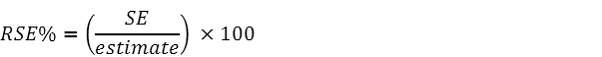5 RSEs for count estimates have been calculated using the Jackknife method of variance estimation. This involves the calculation of 30 'replicate' estimates based on 30 different subsamples of the obtained sample. The variability of estimates obtained from these subsamples is used to estimate the sample variability surrounding the count estimate. 6 The Excel spreadsheets in the Downloads tab contain all the tables produced for this release and the calculated RSEs for each of the estimates. 7 Only estimates (numbers or percentages) with RSEs less than 25% are considered sufficiently reliable for most analytical purposes. However, estimates with larger RSEs have been included. Estimates with an RSE in the range 25% to 50% should be used with caution while estimates with RSEs greater than 50% are considered too unreliable for general use. All cells in the Excel spreadsheets with RSEs greater than 25% contain a comment indicating the size of the RSE. These cells can be identified by a red indicator in the corner of the cell. The comment appears when the mouse pointer hovers over the cell. 8 Another measure is the Margin of Error (MOE), which shows the largest possible difference that could be between the estimate due to sampling error and what would have been produced had all persons been included in the survey with a given level of confidence. It is useful for understanding and comparing the accuracy of proportion estimates. 9 Where provided, MOEs for estimates are calculated at the 95% confidence level. At this level, there are 19 chances in 20 that the estimate will differ from the population value by less than the provided MOE. The 95% MOE is obtained by multiplying the SE by 1.96.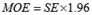Calculation of Standard Error 10 Standard errors can be calculated using the estimates (counts or percentages) and the corresponding RSEs. See What is a Standard Error and Relative Standard Error, Reliability of estimates for Labour Force data for more details. Proportions and Percentages 11 Proportions and percentages formed from the ratio of two estimates are also subject to sampling errors. The size of the error depends on the accuracy of both the numerator and the denominator. A formula to approximate the RSE of a proportion is given below. This formula is only valid when x is a subset of y: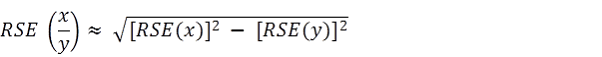Differences 12 The difference between two survey estimates (counts or percentages) can also be calculated from published estimates. Such an estimate is also subject to sampling error. The sampling error of the difference between two estimates depends on their SEs and the relationship (correlation) between them. An approximate SE of the difference between two estimates (x-y) may be calculated by the following formula: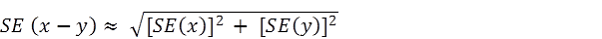13 While this formula will only be exact for differences between separate and uncorrelated characteristics or sub populations, it provides a good approximation for the differences likely to be of interest in this publication. Significance Testing 14 A statistical significance test for a comparison between estimates can be performed to determine whether it is likely that there is a difference between the corresponding population characteristics. The SE of the difference between two corresponding estimates (x and y) can be calculated using the formula shown above in the Differences section. This SE is then used to calculate the following test statistic: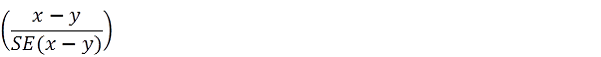15 If the value of this test statistic is greater than 1.96 then there is evidence, with a 95% level of confidence, of a statistically significant difference in the two populations with respect to that characteristic. Otherwise, it cannot be stated with confidence that there is a real difference between the populations with respect to that characteristic. Document Selection These documents will be presented in a new window.